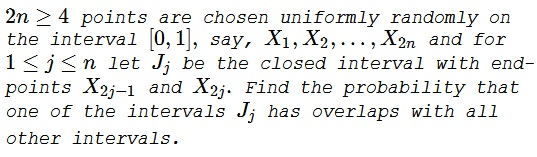# Overlapping Random Intervals

### Problem### Solution

Instead of the unit interval we drop $2n+1$ points on a circle. One, say, $X$ is the starting point for traversing the circle. All other points are labeled in successive pairs $a,b,\ldots.$ By assumption, all cyclic orderings of $2n$ points are equally likely. Given such point placement, start with $X$ and move clockwise until you meet one symbol, say $a,$ for the second time. Turn around, and move counterclockwise until you meet under symbol, say $b,$ twice. Necessarily you have gone beyond $X.$ Now look at the string of symbols between the $b$ and the $a$ at the extremes of this trajectory, but running clockwise, from the (second) $b$ to the (second) $a.$ This string includes $X,$ the other $a$ and the other $b.$ Look at the order in which these three occur. There are just three possibilities:

1. $bXa,$
2. $Xba,$
3. $Xab.$

### Claim

In case 2) and 3), the interval $b$ intersects all others, whereas in case 1) there is no interval that intersects all others.

### Proof

In cases 2) and 3), if $b\text{-interval}$ does not intersect some $c\text{-interval},$ then the relevant symbols occur either as $bccXb$ or $bXccb,$ contradicting the choice of $b$ in the first case and the choice of $a$ in the second.

On the other hand, in case 1), if a $c\text{-interval}$ intersects both the $b\text{-interval}$ and the $a\text{-interval}$, then both $c$ symbols must lie in the string, contradicting the choice of $b.$ This completes the proof.

Since all distributions are equally likely, each of the cases 1)-3) has probability of $\displaystyle \frac{1}{3},$ meaning that the existence of an interval that meets all other intervals is $\displaystyle \frac{2}{3},$ independent of $n.$

### Acknowledgment

The problem and the solution come from

B. Bollobás, The Art of Mathematics: Coffee Time in Memphis, Cambridge U Press, 2006, Problem 102.

B. Bollobás notes that the problem has been solved by Justicz, Scheinerman, and Winkler (Random Intervals, Amer. Math. Monthly, 97 (1990), 881-889.) The above solution is due to Graham Brightwell.

### Geometric Probability